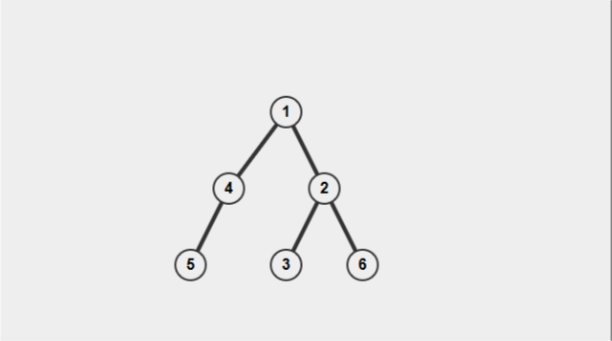Velma and Queries
Tag(s):

Algorithms, Binary search algorithm, Depth-first search, Easy-Medium, Searching algorithm

Problem
Editorial
Analytics

The "Mystery Incorporated" once went for an investigation. Since Scooby and Shaggy always create havoc during investigation, Velma decided to give them a question so as to keep them busy.
Velma gave them a tree consisting of N nodes, rooted at node 1 with a number in each node, and asked them several queries. Each query was of the form u l and they had to find the sum of values of all nodes situated at a depth l and were in the subtree of u.
Since Scooby and Shaggy are dumb, they need your help in solving this problem.

Input

The first line will contain the number of test cases T.
The first line of each test case will contain two integers N and Q (1 ≤ N, Q ≤ 100,000) - the number of nodes and the number of queries.
The second line of each test case will contain N integers a1, a2, ..., aN, where ai (1 ≤ ai ≤ 10,00,000) is the value stored at the ith node.
The next N-1 lines of each test case will contain two integers u and v, meaning that there is an edge connecting u and v.
The next Q lines contains the queries each of the form u and l.

Output

For each query in every test case, print the answer to the query on a single line.

Constraints

• $1 \leq T \leq 5, 1 \leq N, Q \leq 1000, \ 1 \leq a_i \leq 10^6$ in 35% of test cases.
• $1 \leq T \leq 10, 1 \leq N, Q \leq 10^5, \ 1 \leq a_i \leq 10^6$ in 65% of test cases.
SAMPLE INPUT
1
6 5
1 2 3 4 5 6
1 2
2 3
2 6
1 4
4 5
1 1
1 3
2 1
5 3
2 3
SAMPLE OUTPUT
1
14
0
5
9
Explanation

The tree in the test case looks like this.The depths of nodes are:

• Nodes at depth 1: 1
• Nodes at depth 2: 2, 4
• Nodes at depth 3: 3, 5 and 6

For the 2nd query , there are 3 nodes (3, 5, 6) at level 3 which are also in subtree of 1. So the answer would be 3+5+6 = 14.
For the 3rd query, there is only 1 node (1) which is at level 1, but it is not in the subtree of 2. So the answer would be 0.

Time Limit: 2.0 sec(s) for each input file.
Memory Limit: 256 MB
Source Limit: 1024 KB
Marking Scheme: Marks are awarded when all the testcases pass.
Allowed Languages: Bash, C, C++, C++14, Clojure, C#, D, Erlang, F#, Go, Groovy, Haskell, Java, Java 8, JavaScript(Rhino), JavaScript(Node.js), Julia, Kotlin, Lisp, Lisp (SBCL), Lua, Objective-C, OCaml, Octave, Pascal, Perl, PHP, Python, Python 3, R(RScript), Racket, Ruby, Rust, Scala, Swift, Swift-4.1, TypeScript, Visual Basic

CODE EDITOR

Initializing Code Editor...

This Problem was Asked inChallenge Name

October Circuits

OTHER PROBLEMS OF THIS CHALLENGE
• Basic Programming > Implementation
• Math > Combinatorics
• Algorithms > Graphs
• Algorithms > Graphs
• Algorithms > Graphs
• Math > Basic Math